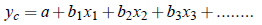Utility of Regression:

In brief, the utility of regression or regression analysis can be understand in the following heads:

1. Estimation of Values: When there is a cause and effect relationship between two variables. The one can be estimated with the help of the other.

2. Determination of the rate of change in the dependent variable for a unit change in the independent variable.

3. Measurement of the errors involved.

4. Measurement of degree and direction of correlation: We can measure the degree and direction of correlation with the help of regression analysis.

Difference Between Correlation and Regression:

The points of difference between correlation and regression are as follows:

1. While correlation analysis tests the closeness with which two (or more) phenomena covary, regression analysis measures the nature and extent of the relation, thus enabling us to make predictions.

2. Correlation analysis has limited applications as it is confined only to the study of relationship between variables; but the regression analysis has much wider application because it also predicts.

3. In the cause-effect relationship, correlation analysis does not consider the concept of dependent and independent variables. On the contrary, in regression analysis one variable is assumed as dependent which the others an independent.

4. In correlation analysis, the correlation coefficient is a measure of degree of co-variability between two variables while the regression analysis deals with a functional relationship between two variables.

Conclusion: A good way of distinguishing between correlation and regression is to consider the correlation phase of study of variable to determine the strength of their relationship and to view regression as that phase which deals with prediction of one variable on the basis of its correlation with other. Naturally correlation should precede regression for if the relationship is not sufficiently strong, there would appear to be no sound basis for prediction.

Kinds of Regression Analysis:

The regression analysis may be classified as:

1. Linear and Curvilinear Regression,

2. Simple and Multiple Regression.

Linear Regression: If the relationship can be represented by a straight line, then it is known as linear regression. In this case the values of the dependent variable changes at a constant rate for a unit change in the values of the independent variable.

Non-liner Regression: When the relationship between variables can be represented by a curve other than straight line, then it is called Non-linear regression.

Simple Regression: If the study is based only on two variables, it is called simple regression.

Multiple Regression: If the study is based on more than two variables, it is called multiple regression.

Multiple Regression Equation:

Let Y = dependent variable, and x1, x2, x3, ...., are independent variables, then multiple regression equation of Y on x1, x2, x3, ...., is written as;where yc = computed value of dependent variable y and b1, b2, b3, .... are multiple regression coefficients. The multiple regression equation expresses the average relationship of the variables and on the basis of this average relationship, an estimate of dependent variable is made. The multiple regression equation shows the simultaneous effect of a number of independent variables.